# How to visualize landscape metrics for local landscapes?

The main goal of this blog post is to present different approaches for visualization of class- and patch-level metrics.

spatial
geocompr
rstats
landscape-ecology

In the past, I wrote a post on how to calculate landscape-level metrics for local landscapes. There, I showed how to divide the categorical input map into a number of smaller areas and calculate selected landscape metrics for each area. The result consists of a regular grid - a number of square polygons, where each polygon contained just one value of a calculated landscape-level metric (e.g., marginal entropy - `ent`). This output is easy to visualize on a map - each polygon can be colored accordingly to its value.

There are, although, two more levels of landscape metrics - the class- and patch-levels. Calculation of class-level metrics returns as many values as unique classes in a local landscape, while the patch-level calculations result in as many values as there are patches1 in each polygon. This makes simple visualizations of class- and patch-level metrics not as straightforward as landscape-level metrics. The main goal of this blog post is to present different approaches for visualization of class- and patch-level metrics.

To reproduce the following results on your own computer, install and attach the packages:

``````library(landscapemetrics)     # landscape metrics calculation
library(raster)               # spatial raster data reading and handling
library(sf)                   # spatial vector data reading and handling
library(dplyr)                # data manipulation
library(tidyr)                # data manipulation
library(tmap)                 # spatial viz
library(geofacet)             # geofacet
library(ggplot2)              # geofacet``````

The first step is to read the input data. Here, we are going to use example data that is already included in the landscapemetrics package .

``````data("augusta_nlcd")
my_raster = augusta_nlcd``````

It is also possible to read any spatial raster file with the `raster()` function, for example `my_raster = raster("path_to_my_file.tif")`2. However, the input file should fulfill two requirements: (1) contain only integer values that represent categories, and (2) be in a projected coordinate reference system. You can check if your file meets the requirements using the `check_landscape()` function, and learn more about coordinate reference systems in the Geocomputation with R book .

Our example data looks like that:

``plot(my_raster)``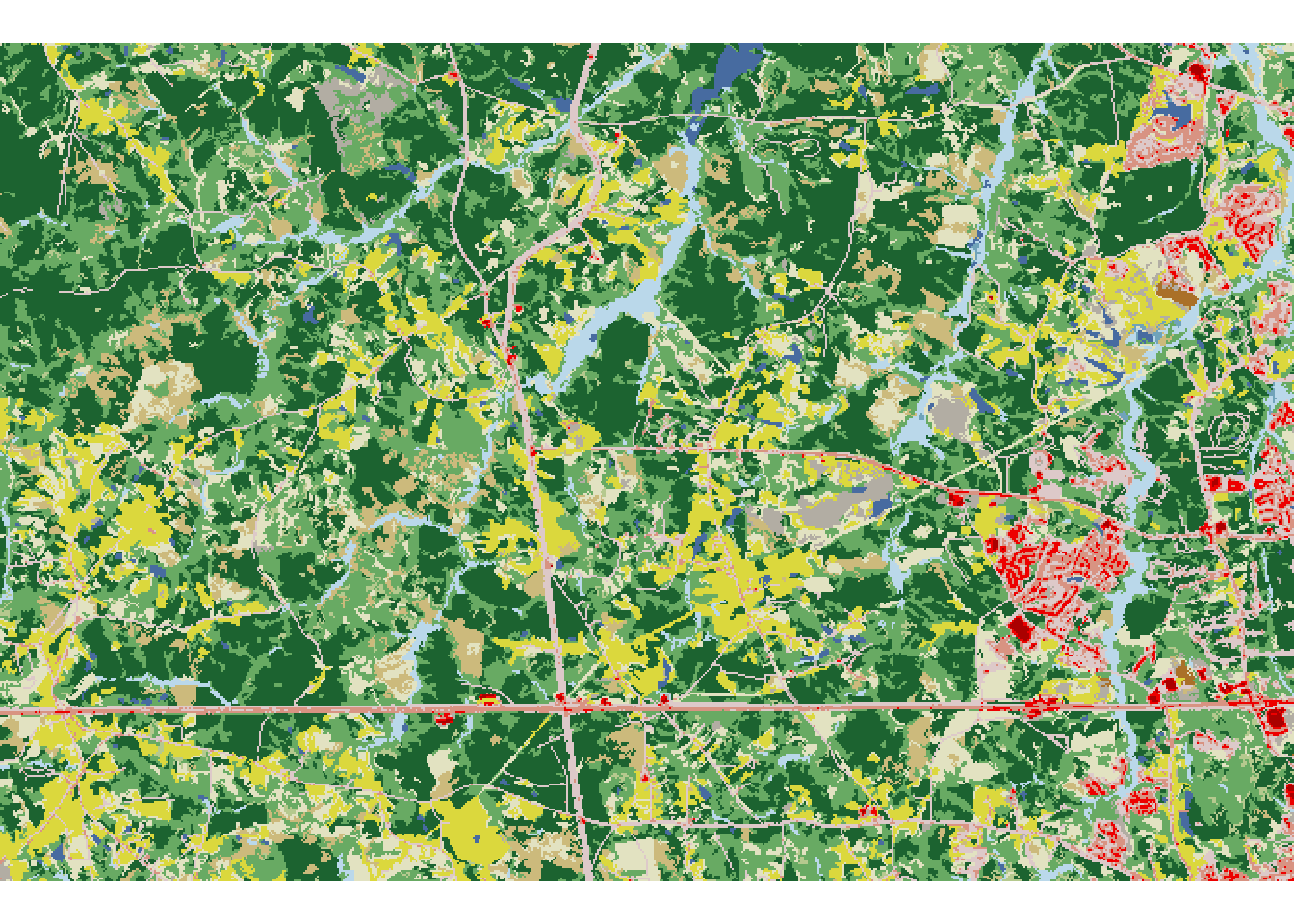# Creating a grid

The next step is to create borders of local landscapes using the `st_make_grid()` function. This function accepts an `sf` object as the first argument, therefore we need to create a new object based on the bounding box of the input raster. Next, we also need to provide a second argument, either `cellsize` or `n`:

• `cellsize` - vector of length 1 or 2 - the side length of each grid cell in map units (usually meters)
• `n` - vector of length 1 or 2 - the number of grid cells in a row/column
``````my_grid_geom = st_make_grid(st_as_sfc(st_bbox(my_raster)), cellsize = 1500)
my_grid_template = st_sf(geom = my_grid_geom)``````

We should also add a unique identification number (`id`) to each grid cell (local landscape).

``my_grid_template\$plot_id = seq_len(nrow(my_grid_template))``

Next, we can overlay the newly created grid on top of our input raster:

``````plot(my_raster)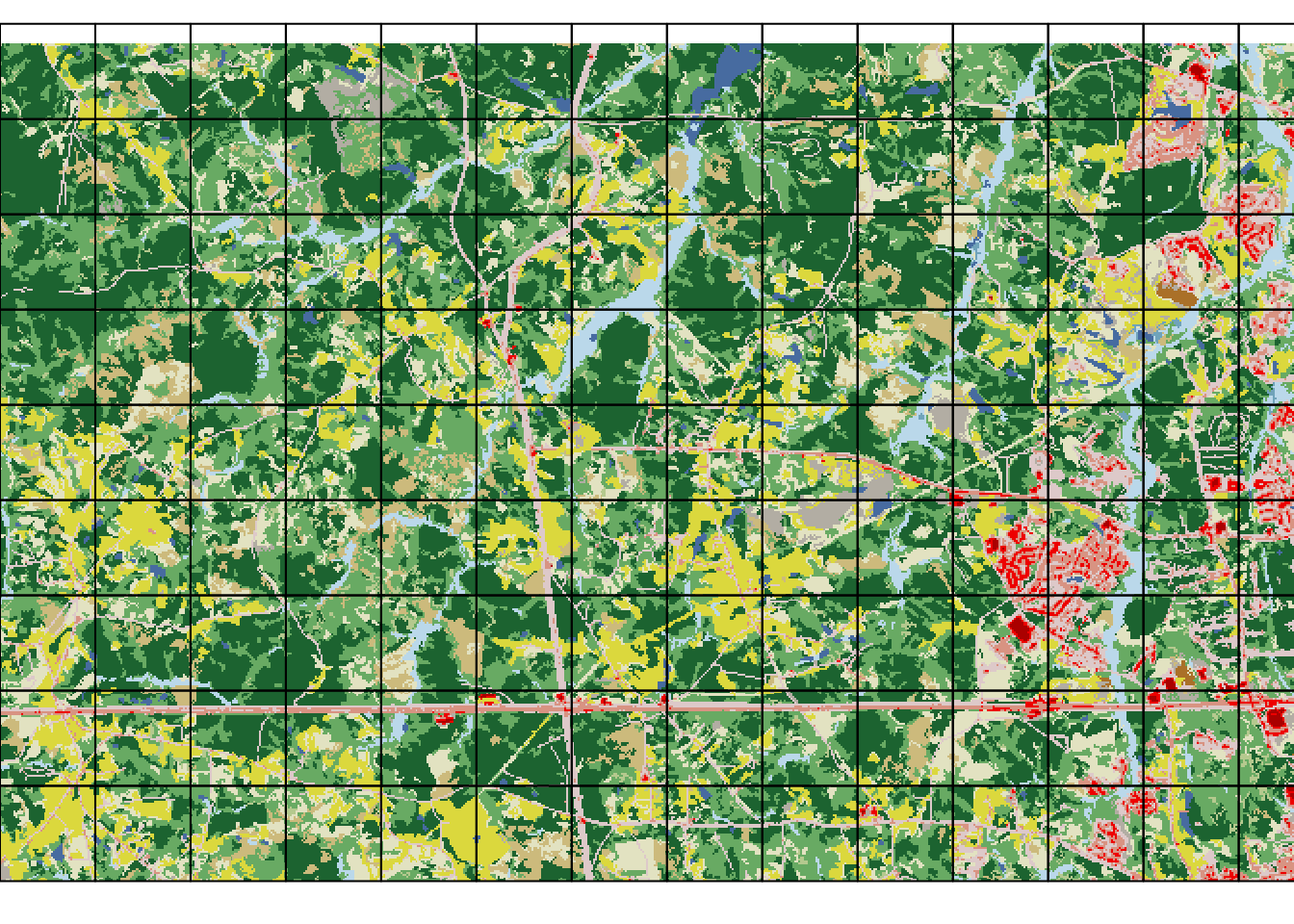Note that some cells cover smaller areas with data than the others.

# Calculating a class-level metric

The calculation of landscape metrics for each cell can be done with the `sample_lsm()` function. It requires an input raster as the first argument, and a grid as the second one3. The function calculates the selected landscape metric independenly for each cell. Next, we can specify which landscape metrics we want to calculate. For this example, we use aggregation index (ai) to be calculated on a class level^[The complete list of the implemented metrics can be obtained with the `list_lsm()` function. Let us know if you are missing some metrics.

``````my_metric1 = sample_lsm(my_raster, my_grid_template,
level = "class", metric = "ai")
my_metric1``````
``````# A tibble: 1,313 × 8
layer level class    id metric value plot_id percentage_inside
<int> <chr> <int> <int> <chr>  <dbl>   <int>             <dbl>
1     1 class    11    NA ai      83.3       1               100
2     1 class    21    NA ai      34.5       1               100
3     1 class    22    NA ai      23.0       1               100
4     1 class    31    NA ai      86.8       1               100
5     1 class    41    NA ai      63.9       1               100
6     1 class    42    NA ai      73.2       1               100
7     1 class    43    NA ai      39.5       1               100
8     1 class    52    NA ai      43.1       1               100
9     1 class    71    NA ai      66.9       1               100
10     1 class    81    NA ai      85.8       1               100
# … with 1,303 more rows``````

Each row in the `my_metric` object corresponds to one calculated value of ai, while the `plot_id` column specifies to which grid cell the results are related 4. Because there are several classes in each cell, there are also several ai values for each cell present. Next, we can connect spatial grid (`my_grid_template`) with the calculation results (`my_metric1`) using the `left_join()` function:

``my_grid1 = left_join(my_grid_template, my_metric1, by = "plot_id")``

# Vizualizing the class-level results

For the class-level results, each local landscape has as many values as unique classes belong to it. It prevents us from creating a single comprehensive map, but, on the other hand, allows for some other visualizations.

## Subsets

The most basic approach is to create a map just for a selected class, which can be done with the `subset()` function:

``````my_grid1_class1 = subset(my_grid1, class == 11)
plot(my_grid1_class1["value"])``````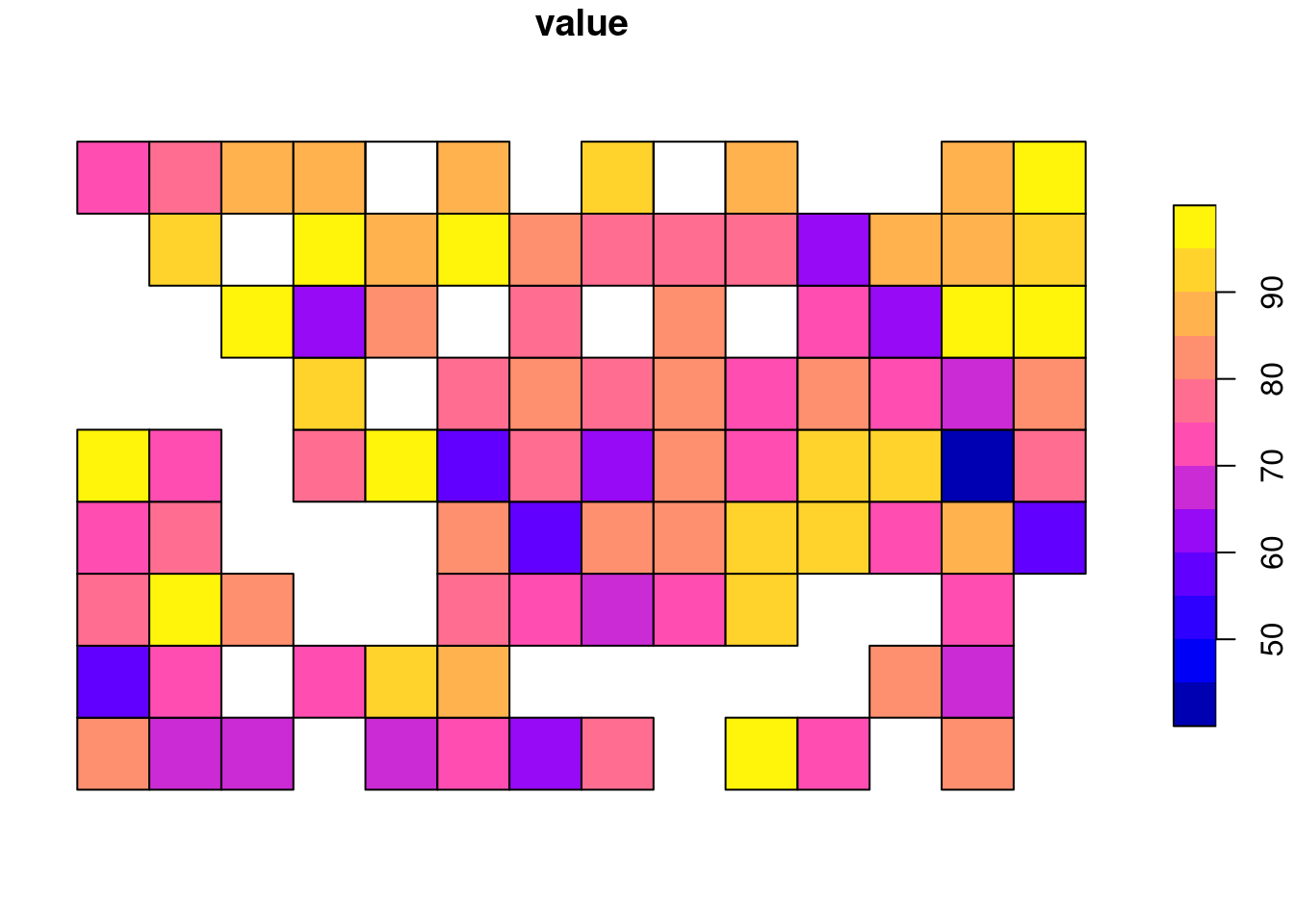The above plot shows the distribution of ai values for class 11.

## Map facets

It is also possible to quickly visualize all of the subsets at the same time with the tmap package .

``````tm_shape(my_grid1) +
tm_polygons("value", style = "cont", title = "ai") +
tm_facets(by = "class", free.coords = FALSE)``````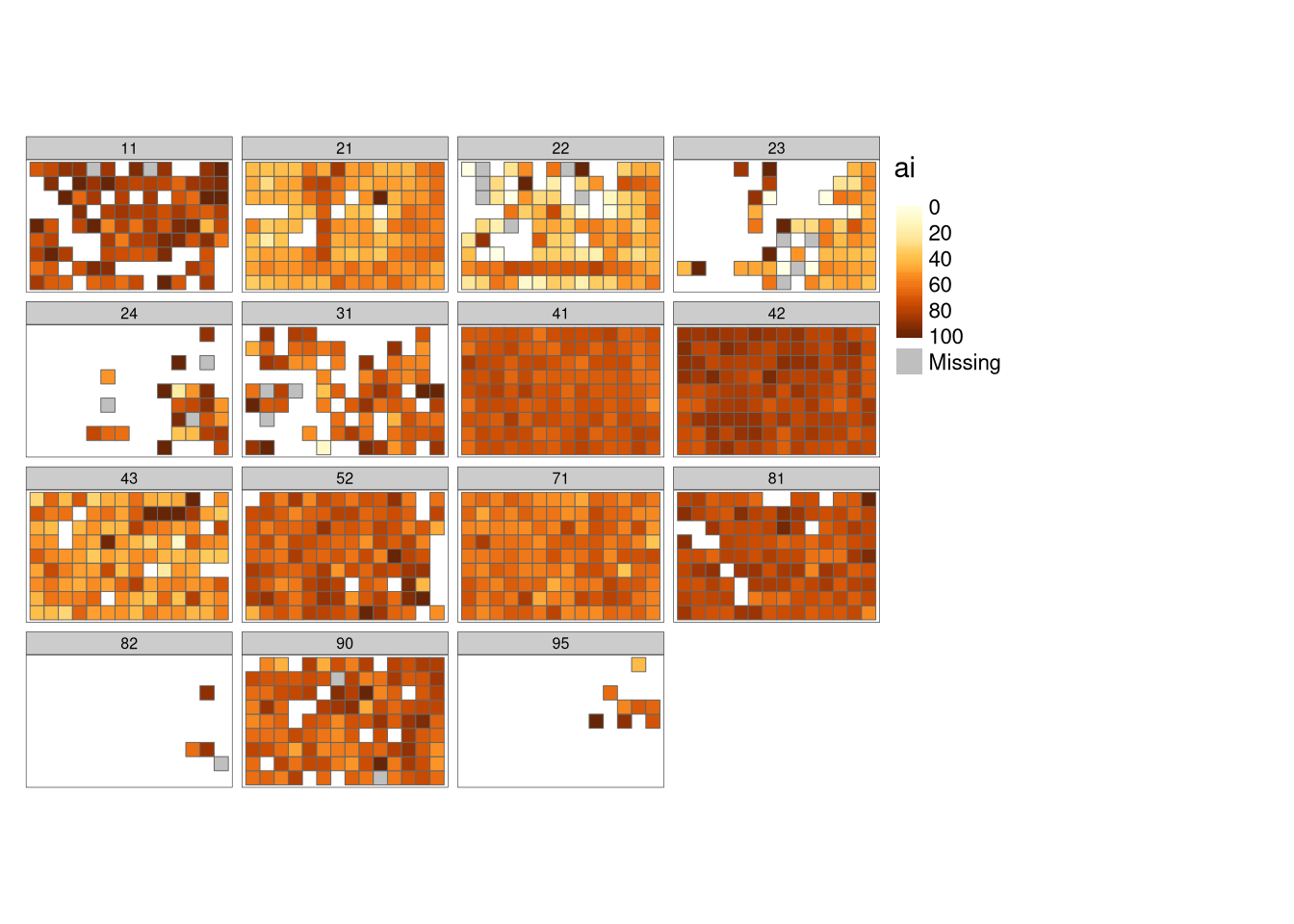The result here contains a separate panel for each unique class in our dataset, while colors represent different AI values.

## geofacet

As our local landscapes are made of a regular grid, we can also test some less traditional visualizations. One possibility is to use the geofacet package - it allows to create many regular plots (such as histograms, scatterplots, boxplots, etc.), but arrange them spatially. Visit the package website at https://github.com/hafen/geofacet to find more examples.

The first step here is to create a plotting grid with `grid_auto()`:

``grd = grid_auto(my_grid_template, names = "plot_id")``

Next, we can create a plot with the ggplot2 syntax adding `facet_geo(~plot_id, grid = grd)` to it:

``````ggplot(my_grid1, aes(as.factor(class), value, fill = as.factor(class))) +
geom_col() +
facet_geo(~plot_id, grid = grd) +
labs(x = NULL, y = "ai", fill = "Class") +
theme(axis.text.x = element_blank(),
axis.ticks.x = element_blank(),
strip.background = element_blank(),
strip.text.x = element_blank())``````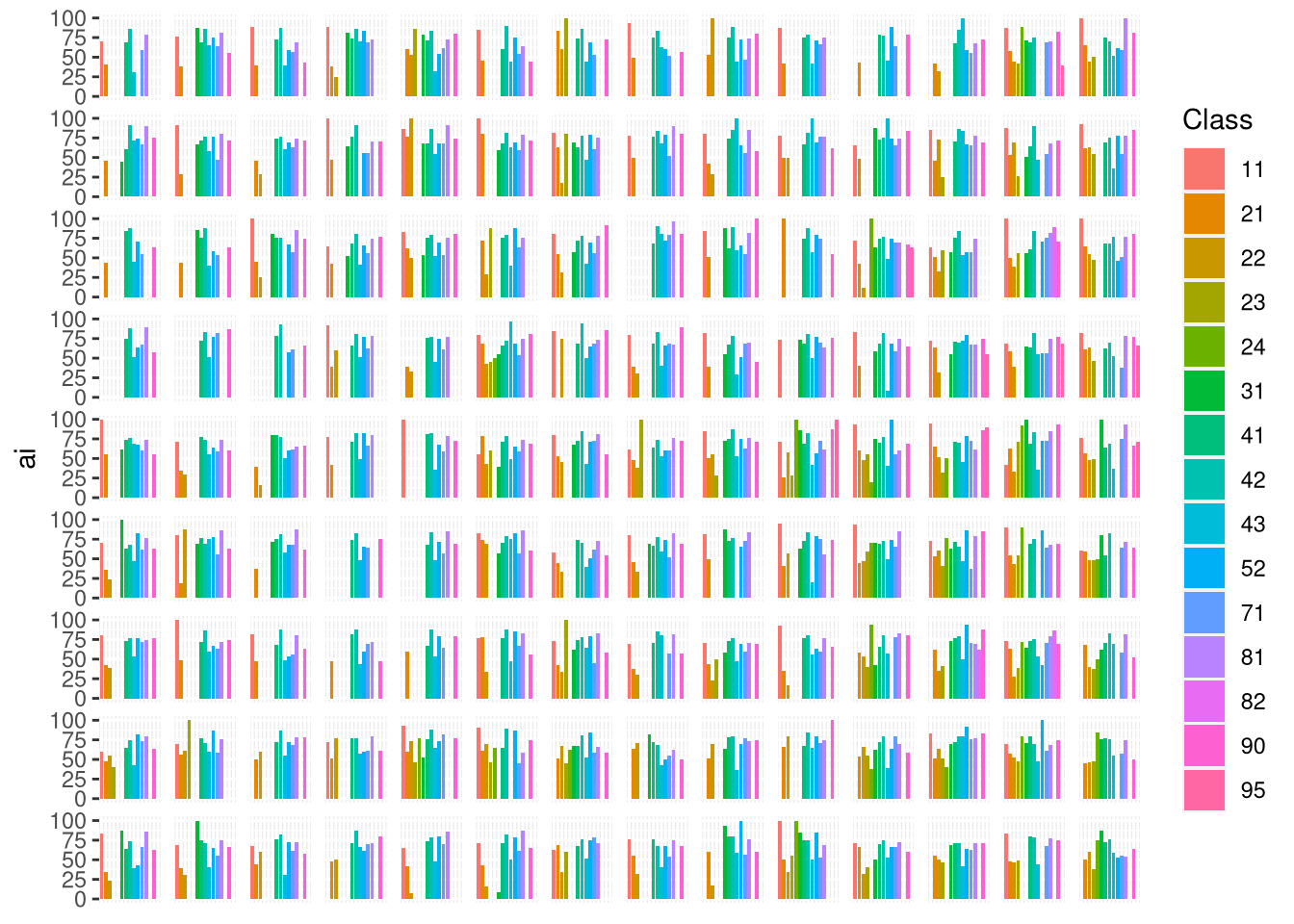Here, we can see a visualization that consists of many separate bar plots. Each bar plot represents ai values for each local landscape.

# Calculating a patch-level metric

The calculation of patch-level metrics can also be done with the `sample_lsm()` function. In this example, we use euclidean nearest-neighbor distance (enn) - a metric that can only be calculated on a patch level.

``````my_metric2 = sample_lsm(my_raster, my_grid_template,
level = "patch", metric = "enn")
my_metric2``````
``````# A tibble: 10,479 × 8
layer level class    id metric value plot_id percentage_inside
<int> <chr> <int> <int> <chr>  <dbl>   <int>             <dbl>
1     1 patch    11     1 enn    201.        1               100
2     1 patch    11     2 enn    201.        1               100
3     1 patch    11     3 enn    495.        1               100
4     1 patch    21     4 enn    433.        1               100
5     1 patch    21     5 enn     67.1       1               100
6     1 patch    21     6 enn     67.1       1               100
7     1 patch    21     7 enn     67.1       1               100
8     1 patch    21     8 enn     67.1       1               100
9     1 patch    21     9 enn     60         1               100
10     1 patch    21    10 enn     60         1               100
# … with 10,469 more rows``````

The result contains a large number of rows, where each row is related to a unique combination of a patch, a class, and a grid cell. We can connect spatial grid (`my_grid_template`) with the calculation results (`my_metric2`) again using the `left_join()` function:

``my_grid2 = left_join(my_grid_template, my_metric2, by = "plot_id")``

# Vizualizing the patch-level results

Visualization of the patch-level results could be the most challenging to create. Here, each local landscape can have as little as one value (a single large patch) and as many values as cells. Patches also can be related to just a few or to many different classes.

## One patch map

The most straightforward approach here is to use the `spatialize_lsm()` function, which calculates a selected patch-level metric for a given raster, and returns a new raster with the metric values.

``my_metric_r_all = spatialize_lsm(my_raster, level = "patch", metric = "enn")``

The output of `spatialize_lsm()` is a nested list. Each list element is used to represent different input layers (e.g., land cover data for different years), while each sub-list element relates to the calculated metric (you are able to calculate many metrics at the same time).

``plot(my_metric_r_all\$layer_1\$lsm_p_enn)``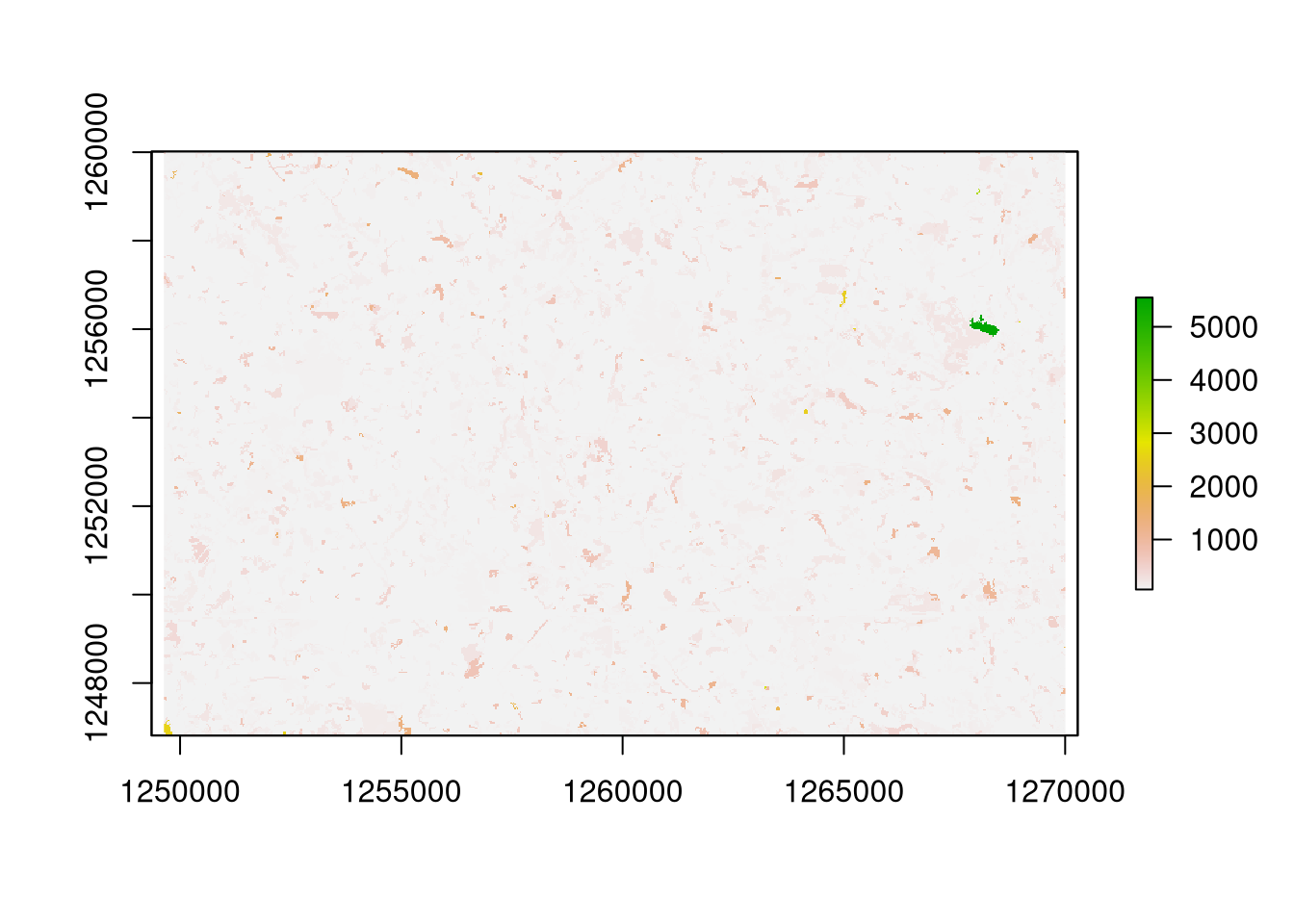The `spatialize_lsm()` function is fine when we need to present patch-level values for a whole raster. However, if we have many local landscapes (or sub-rasters) then we need to repeat the `spatialize_lsm()` calculations for each area.

## Patch maps

This can be done with the help of the following code.

``````patch_raster = function(my_raster, my_grid){
result = vector(mode = "list", length = nrow(my_grid))
for (i in seq_len(nrow(my_grid))){
my_small_raster = crop(my_raster, my_grid[i, ])
result[[i]] = spatialize_lsm(my_small_raster,
level = "patch", metric = "enn")
}
return(result)
}

my_metric_r = patch_raster(my_raster, my_grid_template)
my_metric_r = unlist(my_metric_r)
names(my_metric_r) = NULL
my_metric_r = do.call(merge, my_metric_r)``````

In it, we go through each grid cell, crop a raster to its extent, and calculate our metric of interest. The result is a large list consisting of many separate small rasters, that we can combine to get a full-size raster in return with `do.call()` and `merge()`.

``````plot(my_metric_r)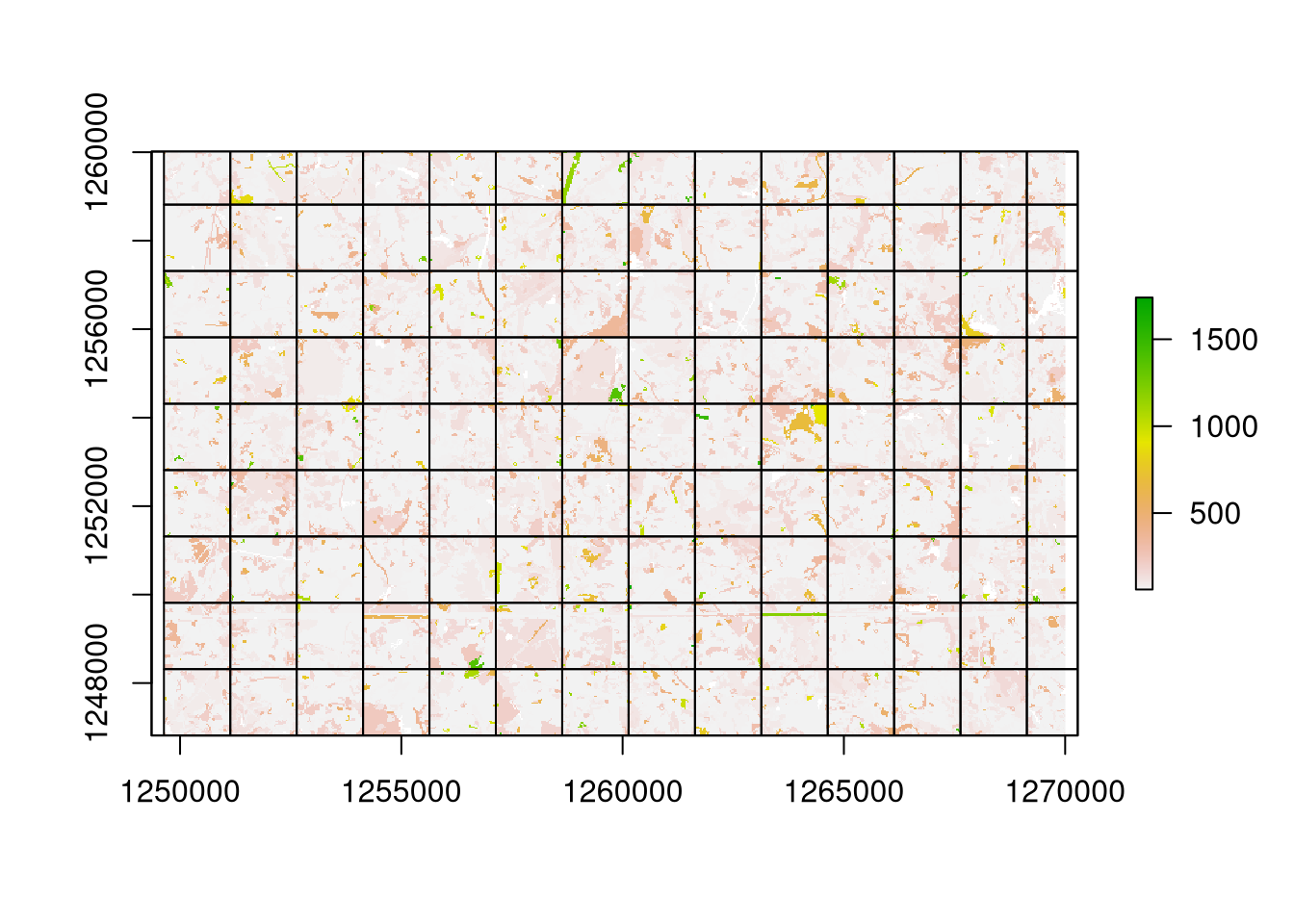The resulting visualization looks much different from the previous one. In it, each local landscape is treated independently, therefore, a patch belonging to many grid cells is split into many patches.

# Summary

The landscapemetrics package, together with many other open-source R packages, allows for spatial visualizations of class- and patch-level metrics. The class-level metrics can be presented independently for each class, or as a geofacet plot, while patch-level metrics might be visualized in combination with the `spatialize_lsm()` function. It is also worth to mention that patch-level metrics can also use the geofacet package; however, this could work best when the number of local landscapes is relatively small. Otherwise, the resulting plot could be hard to read. To learn more about landscape metrics and the landscapemetrics package, visit https://r-spatialecology.github.io/landscapemetrics/ and http://dx.doi.org/10.1111/ecog.04617.

# Acknowledgement

Many thanks to Maximilian H.K. Hesselbarth for reading and improving a draft of this blog post.

## References

Hafen, Ryan. 2020. Geofacet: ’Ggplot2’ Faceting Utilities for Geographical Data. https://CRAN.R-project.org/package=geofacet.
Hesselbarth, Maximilian H. K., Marco Sciaini, Kimberly A. With, Kerstin Wiegand, and Jakub Nowosad. 2019. “Landscapemetrics: An Open-Source r Tool to Calculate Landscape Metrics.” Ecography 42: 1648–57.
Lovelace, Robin, Jakub Nowosad, and Jannes Muenchow. 2019. Geocomputation with R. CRC Press.
Tennekes, Martijn. 2018. tmap: Thematic Maps in R.” Journal of Statistical Software 84 (6): 1–39. https://doi.org/10.18637/jss.v084.i06.
Wickham, Hadley. 2016. Ggplot2: Elegant Graphics for Data Analysis. Springer-Verlag New York. https://ggplot2.tidyverse.org.

## Footnotes

1. A patch is a group of adjacent cells of the same category.↩︎

2. Currently landscapemetrics accepts also objects from the terra and stars packages.↩︎

3. This function also allows for many more possibilities, including specifying a 2-column matrix with coordinates, SpatialPoints, SpatialLines, SpatialPolygons, sf points or sf polygons as the second argument. You can learn all of the valid options using `?sample_lsm`.↩︎

4. To learn more about the structure of the output read the Efficient landscape metrics calculations for buffers around sampling points blog post.↩︎

## Citation

BibTeX citation:
``````@online{nowosad2022,